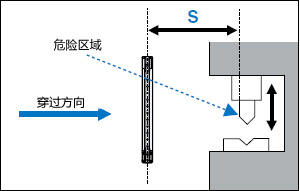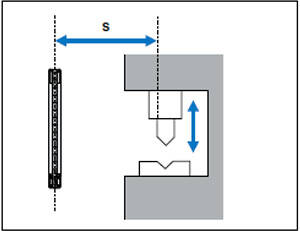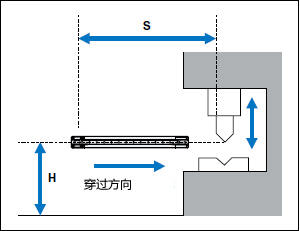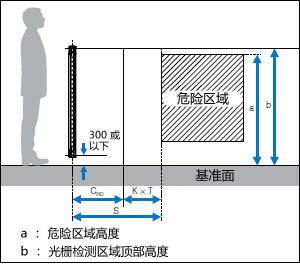• TOP
•  >
• 选购安全设备前| 光栅的安装与安全距离

# 光栅的安装与安全距离

## 按照ISO 13855 标准计算安全距离

### 与接近方向垂直的方向S = K × T + C　40 < d ≦ 70
K = 1600 mm/s（接近速度[ 假定为人的步行速度]）
T = 机器停止所需的最长时间 + 光栅响应时间
C = 850 mm（穿过距离[ 与人手臂标准长度相符的值]）

S=K × T + 8(d - 14) d ≦ 40
K = 2000 mm/s（接近速度[ 假定手的穿过速度]）
T = 机器停止所需的最长时间 + 光栅响应时间
d = 光栅检测能力

### 机器停止所需的最长时间与安全距离之间的关系

T = 机器停止所需的最长时间 + 光栅响应时间（ON  OFF）

S = 2000 mm/s ×（ 机器停止所需的最长时间 + 0.0069 s） + C

## 基本计算示例

### 与接近方向垂直的方向： GL-R 系列• S: 最小距离（mm；见下图） ≥ 100 mm
• K: 从基于人体接近速度（mm/s）得出的数据中提取的参数
• T: 整个系统停止性能（s） T = t1（GL-R 系列最长响应时间） + t2（机器停止所需的最长时间）
• C:穿过距离（mm）
当 d ≤ 40: 8 × (d - 14) , C ≥ 0
当 40 < d ≤ 70: 850
• d: GL-R 系列的检测能力（mm）

K = 2000 mm/s
t1（GL-R60H 响应时间） = 0.0157 s
t2（机器停止所需的最长时间） = 0.1 s
C = 8 × (25 - 14) = 88 mm

S = K × T + C = 2000 ×（0.1157）+ 88 = 319.4mm

（检测能力d = 45 mm 且光轴数为8）时

K = 1600 mm/s
t1（GL-R08L 响应时间） = 0.0069 s
t2（机器停止所需的最长时间） = 0.1 s
C = 850 mm

S = K × T + C = 1600 × (0.1069) + 850 = 1021.04 mm

### 与接近方向平行的方向： GL-R 系列• S: 最小距离（见下图）
• K: 从基于人体接近速度（mm/s）得出的数据中提取的参数
• T: 整个系统停止性能（s）
T = t1（GL-R 系列最长响应时间） + t2（机器停止所需的最长时间）
• C: 穿过距离（mm）
C = 1200 - 0.4H with C ≥ 850 mm
H: 基准面上方检测区域高度（mm）
15 (d - 50) ≤ H ≤ 1000 H ≥ 0

（检测能力d = 45 mm 且光轴数为30）时

K = 1600 mm/s
t1（GL-R30L 响应时间） = 0.0105 s
t2（机器停止所需的最长时间） = 0.1 s
H = 200 mm
C = 1200 - 0.4 × 200 = 1120 mm
S = K × T + C = 1600 × (0.0105 + 0.1) + 1120 mm = 1296.8 mm

### 通过绕过检测区域顶部来接近危险: GL-R 系列• S: 最小距离（mm；见右图），S ≥ 100 mm
• K: 从基于人体接近速度（mm/s）得出的数据中提取的参数
S (mm) K (mm)
100 ≤ S ≤ 500 2000
500 < S 1600*1 不包括检测区域顶部低于900 mm 的情况，因为在这种情况下，无法采取充分的保护措施来避免规避和跨越行为。
*2 如果检测区域底部距离基准面的高度超过300 mm，则无法采取充分的保护措施来防止人员从检测区域下方通过并接近危险。

（检测能力d = 25 mm，光轴数为60，检测高度为1180 mm）时

a（危险区域高度）= 1400 mm
b（光栅检测区域顶部高度）= 1180 + 300 = 1480 mm

（由于b 值为1480 mm，介于1400 和1600 之间，在这种情况下，b 值取1400。）
• K = 1600 mm/s
• t1（GL-R60H 响应时间） = 0.0157 s
• t2（机器停止所需的最长时间） = 0.1 s
S = K × T + CRO = 1600 × (0.1157) + 850 = 1035.12 mm
（该值大于按照“与接近方向垂直的方向：GL-R 系列” 算出的S 值）

（检测能力d = 25 mm，光轴数为80，检测高度为1580 mm）时

a（危险区域高度）= 1400 mm
b（光栅检测区域顶部高度）= 1580 + 300 = 1880 mm（由于b 值为1880 mm，介于1800 和2000 之间，在这种情况下，b 值取1800。）
• K = 2000 mm/s
• t1（GL-R80H 响应时间）= 0.0192 s
• t2（机器停止所需的最长时间） = 0.1 s
S = K × T + CRO = 2000 × (0.1192) + 0 = 238.4 mm
（该值小于按照“与接近方向垂直的方向：GL-R 系列” 算出的S值）